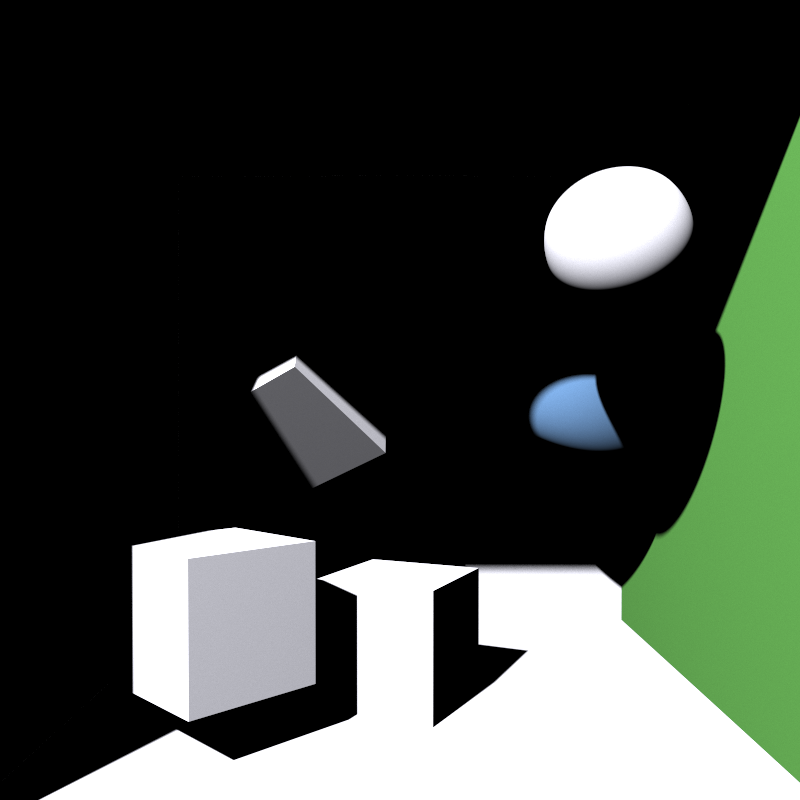## Importance Sampled Direct Lighting

Takua Render now has correct, fully working importance sampled direct lighting, supported for any type of light geometry! More importantly, the importance sampled direct lighting system is now fully integrated with the overall GI pathtracing integrator.

A naive, standard pathtracing implementation shoots out rays and accumulates colors until a light source is reached, upon which the total accumulated color is multiplied by the emittance of the light source and added to the framebuffer. As a result, even the simplest pathtracing integrator does account for both the indirect and direct illumination within a scene, but since sampling light sources is entirely dependent on the BRDF at each point, correctly sampling the direct illumination component in the scene is extremely inefficient. The canonical example of this inefficiency is a scene with a single very small, very intense, very far away light source. Since the probability of hitting such a small light source is so small, convergence is extremely slow.

To demonstrate/test this property, I made a simple test scene with an extremely bright sun-like object illuminating the scene from a huge distance away: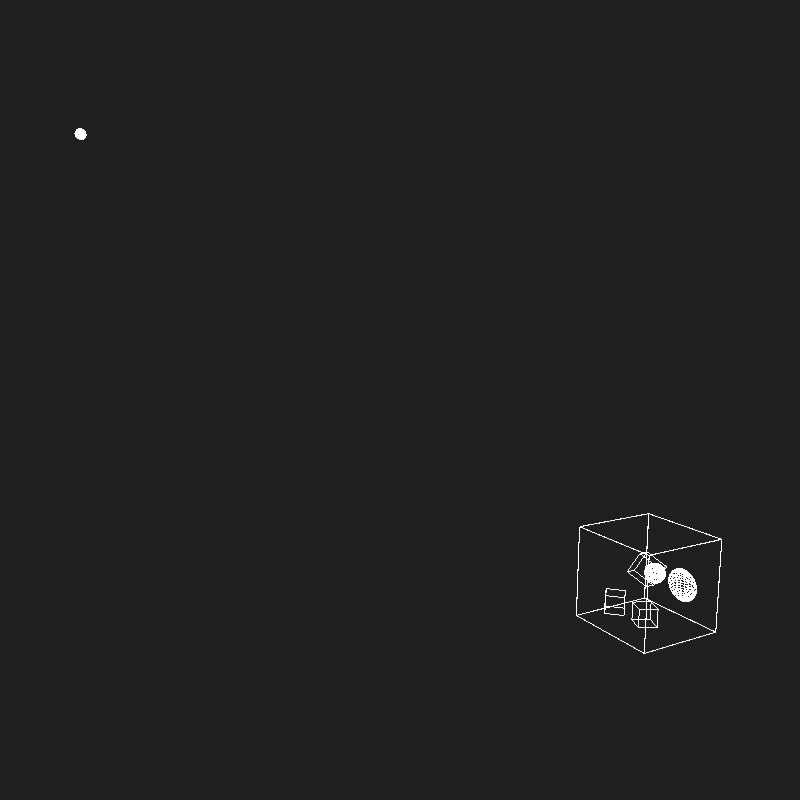Using naive pathtracing without importance sampled direct lighting produces an image like this after 16 samples per pixel: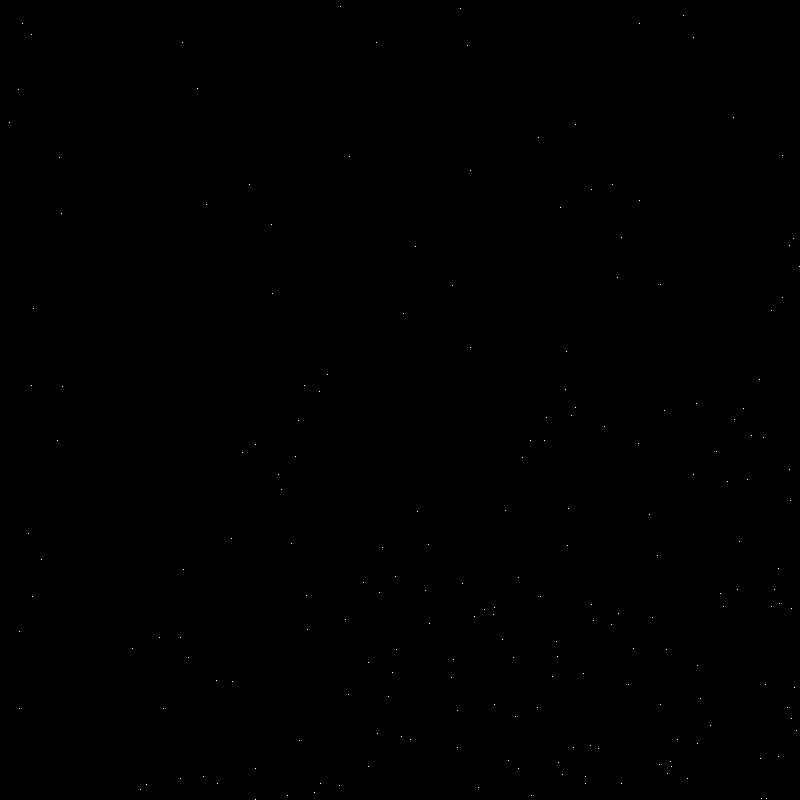Mathematically, the image is correct, but is effectively useless since so few contributing ray paths have actually been found. Even after 5120 samples, the image is still pretty useless:Instead, a much better approach is to accumulate colors just like before, but not bother waiting until a light source is hit by the ray path through pure BRDF sampling to multiply emittance. Instead, at each ray bounce, a new indirect ray is generated via the BRDF like before, AND to generate a new direct ray towards a randomly chosen light source via multiple importance sampling and multiply the accumulated color by the resultant emittance. Multiple importance sampled direct lighting works by balancing two different sampling strategies: sampling by light source and sampling by BRDF, and then weighting the two results with some sort of heuristic (such as the power heuristic described in Eric Veach’s thesis).

Sampling by light source is the trickier part of this technique. The idea is to generate a ray that we know will hit a light source, and then weight the contribution from that ray by the probability of generating that ray to remove the bias introduced by artificially choosing a ray direction. There’s a few good ways to do this: one way is to generate an evenly distributed random point on a light source as the target for the direct lighting ray, and then weight the result using the probability distribution function with respect to surface area, transformed into a PDF with respect to solid angle.

Takua Render at the moment uses a slightly different approach, for the sake of simplicity. The approach I’m using is similar to the one described in my earlier post on the topic, but with a disk instead of a sphere. The approach works like this:

1. Figure out a bounding sphere for the light source
2. Construct a ray from the point to be lit to the center of the bounding sphere. Let’s call the direction of this ray D.
3. Find a great circle on the bounding sphere with a normal N, such that N is lined up exactly with D.
4. Move the great circle along its normal towards the point to be lit by a distance of exactly the radius of the bounding sphere
5. Treat the great circle as a disk and generate uniformly distributed random points on the disk to shoot rays towards.
6. Weight light samples by the projected solid angle of the disk on the point being lit.

Alternatively, the weighting can simply be based on the normal solid angle instead of the projected solid angle, since the random points are chosen with a cosine weighted distribution.

The nice thing about this approach is that it allows for importance sampled direct lighting even for shapes that are difficult to sample random points on; effectively, the problem of sampling light sources is abstracted away, at the cost of a slight loss in efficiency since some percentage of rays fired at the disk have to miss the light in order for the weighting to remain unbiased.

I also started work on the surface area PDF to solid angle PDF method, so I might post about that later too. But for now, everything works! With importance sampled direct lighting, the scene from above is actually renderable in a reasonable amount of time. With just 16 samples per pixel, Takua Render now can generate this image: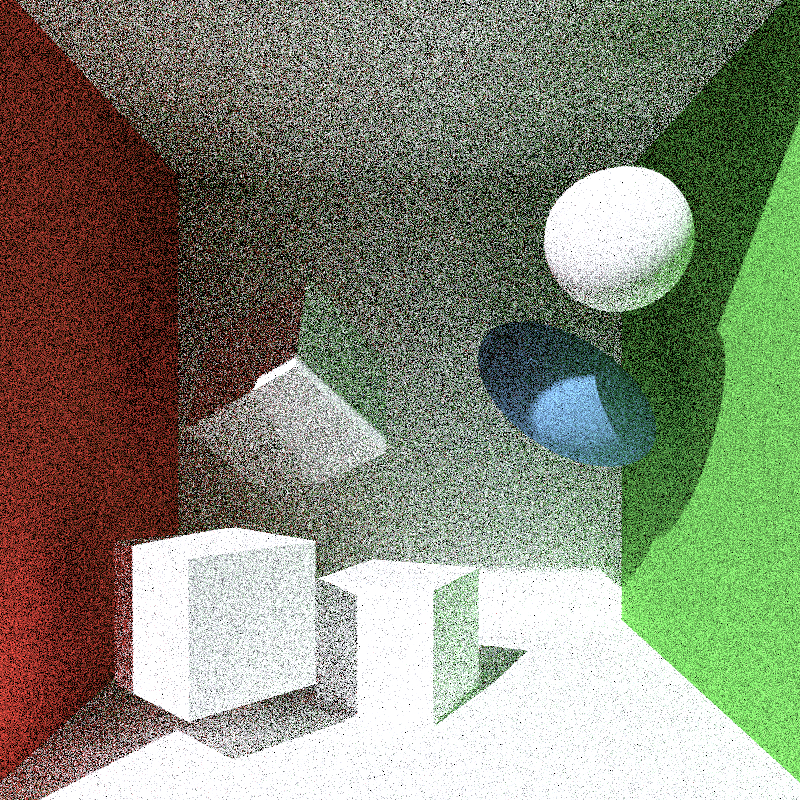…and after 5120 samples per pixel, a perfectly clean render: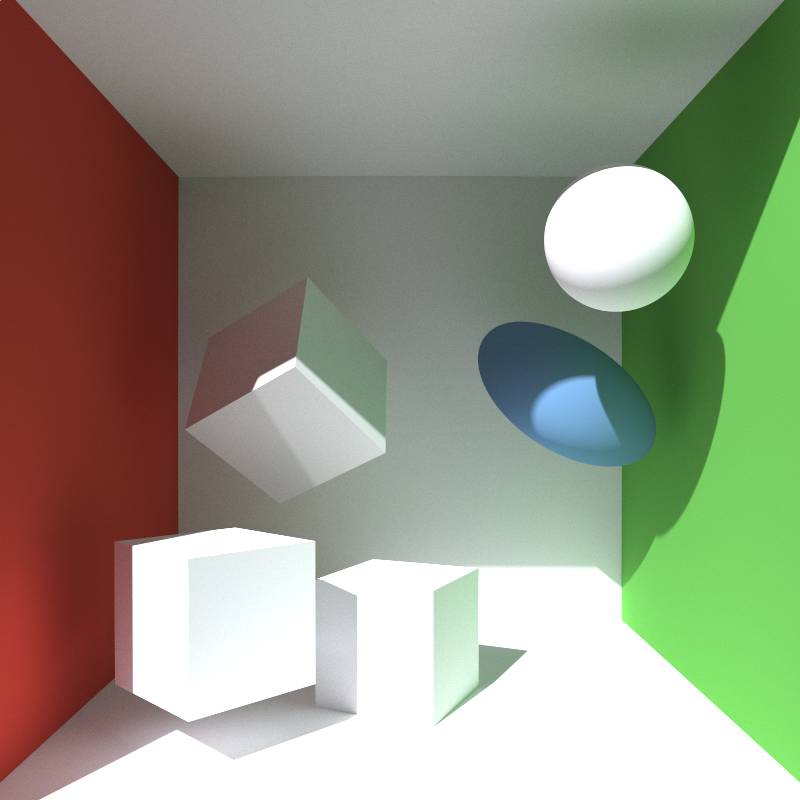The other cool thing about this scene is that most of the scene is actually being lit through pure indirect illumination. With only direct illumination and no GI, the render looks like this: